# How to Sum Values of Cells Occurring at the Intersection of Ranges Excel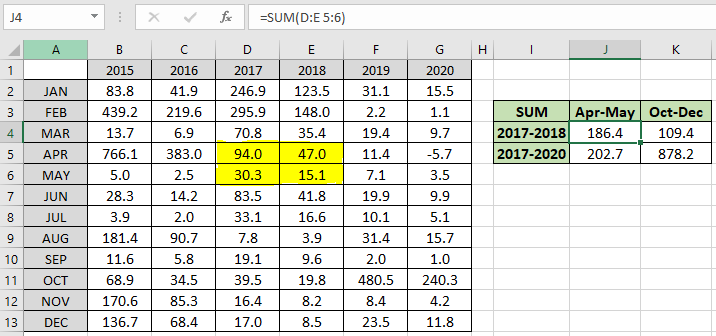In this article, we will learn How to Sum Values of Cells Occurring at the Intersection of Ranges Excel

Scenario:

When working with a long dataset. Sometimes we need to find the sum of cells which occur at the intersection of given rows and columns. For example finding the sum of sales for two months in given years. We can indicate the rows and columns in the SUM formula to get the result. Let's understand how to use the SUM function to get the intersection sum.

Formula

We can use SUM function with different usages. Here we use the SUM function to sum given COLUMNS and ROWS.

Formula syntax:

 =SUM (col1:col2  row1:row2)

col1 : start column Index

col2 : end column Index

row1 : start row Index

row2 : end row Index

Example :

All of these might be confusing to understand. Let's understand how to use the function using an example. Here we have 6 years sales data with given months. Now we want the sum of Apr-May month for the 2017-2018 years.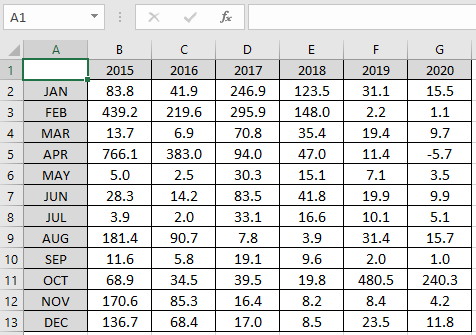Now we know the col1 will be column D (2017) and col2 will be column E (2018). whereas row1 will be row 5(Apr) and row2 will be row 6 (May). now use these in the formula stated below.

Use the formula:

 =SUM(D:E 5:6)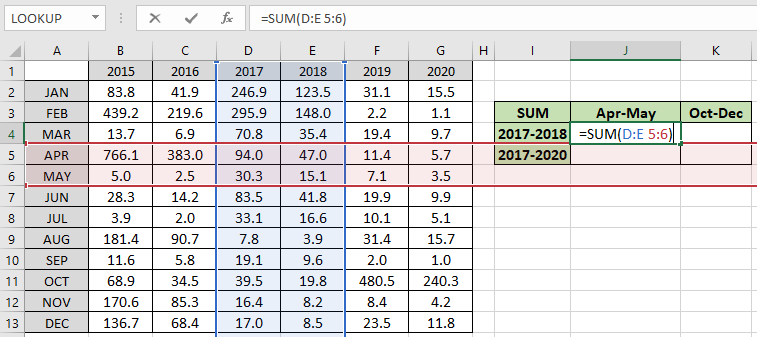You can confirm the intersection part with highlighted colors. Press Enter to get the results.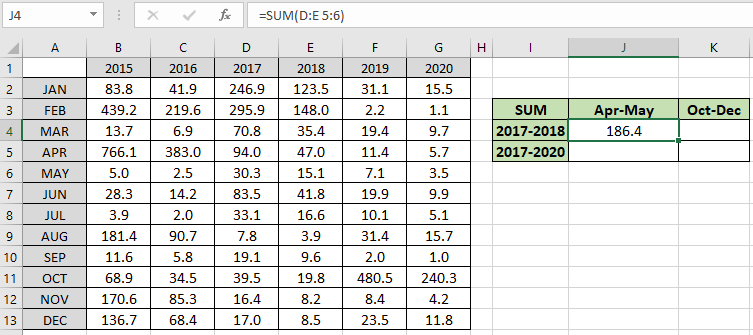As you can see the sum of 94.0 + 47.0 + 30.3 + 83.5 = 186.4. Now you can find the sum of any values on the intersection of any number of rows and columns. Similarly for given years and months we can find the sum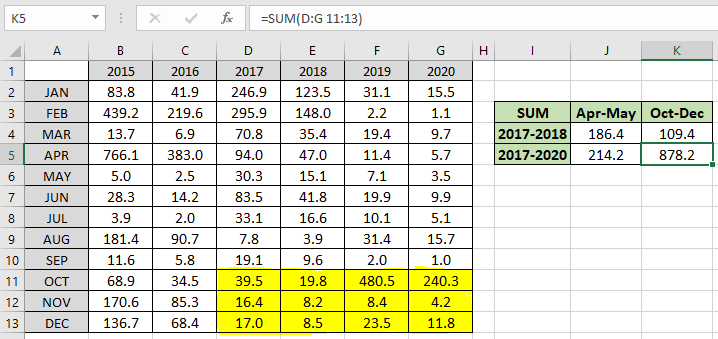As you can see the yellow shaded part sum is 878.2 . Now you must be wondering if there are two intersection points. Then how can we get the sum of those two intersections? Just use a comma between the two inputs. For example getting the sum of Jan - feb month for year 2015-2016 & Nov -Dec month for year 2018 - 2019. Use the formula to get the result.

Use the formula:

 =SUM(B:C 2:3, E:F 12:13)Press Enter to get the result.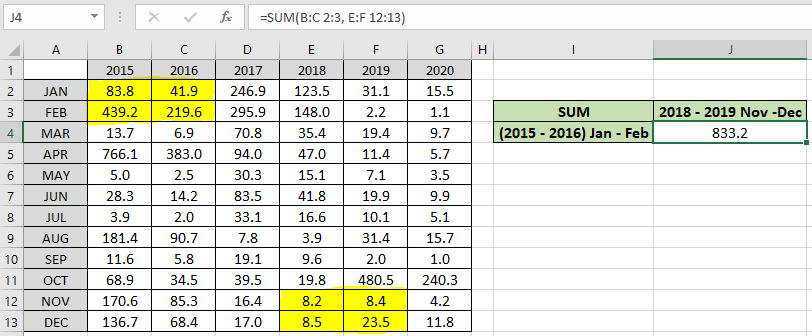As you can see the sum of the yellow shaded part comes out to be 833.2 .

Notes :

1. Use the space character for the intersection of rows and columns.
2. Use the comma character for the 2 intersectional results.

Hope this article about How to Sum Values of Cells Occurring at the Intersection of Ranges Excel is explanatory. Find more articles on summing formulas and related Excel formulas here. If you liked our blogs, share it with your friends on Facebook. And also you can follow us on Twitter and Facebook. We would love to hear from you, do let us know how we can improve, complement or innovate our work and make it better for you. Write to us at info@exceltip.com.

Related Articles

How to use the SUMPRODUCT function in Excel: Returns the SUM after multiplication of values in multiple arrays in excel.

SUM if date is between : Returns the SUM of values between given dates or period in excel.

Sum if date is greater than given date: Returns the SUM of values after the given date or period in excel.

2 Ways to Sum by Month in Excel: Returns the SUM of values within a given specific month in excel.

How to Sum Multiple Columns with Condition: Returns the SUM of values across multiple columns having condition in excel

How to use wildcards in excel : Count cells matching phrases using the wildcards in excel

Popular Articles :

How to use the IF Function in Excel : The IF statement in Excel checks the condition and returns a specific value if the condition is TRUE or returns another specific value if FALSE.

How to use the VLOOKUP Function in Excel : This is one of the most used and popular functions of excel that is used to lookup value from different ranges and sheets.

How to Use SUMIF Function in Excel : This is another dashboard essential function. This helps you sum up values on specific conditions.

How to use the COUNTIF Function in Excel : Count values with conditions using this amazing function. You don't need to filter your data to count specific values. Countif function is essential to prepare your dashboard.

1.